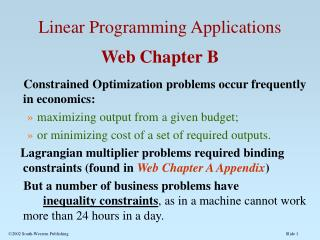DownloadDownload PresentationLinear Programming Applications Web Chapter B

Linear Programming Applications Web Chapter B

Download PresentationLinear Programming Applications Web Chapter B

- - - - - - - - - - - - - - - - - - - - - - - - - - - E N D - - - - - - - - - - - - - - - - - - - - - - - - - - -
Presentation Transcript

1. Linear Programming ApplicationsWebChapter B Constrained Optimization problems occur frequently in economics: • maximizing output from a given budget; • or minimizing cost of a set of required outputs. Lagrangian multiplier problems required binding constraints (found in Web Chapter A Appendix) But a number of business problems have inequality constraints, as in a machine cannot work more than 24 hours in a day. 2002 South-Western Publishing

2. Profit Maximization Problem Using Linear Programming • Constraints of production capacity, time, money, raw materials, budget, space, and other restrictions on choices. • These constraints can be viewed as inequality constraints, or . • A "linear" programming problem assumes a linear objective function, and a series of linear inequality constraints

3. Linearity implies: 1. constant prices for outputs (as in a perfectly competitive market). 2. constant returns to scale for production processes. 3. Typically, each decision variable also has a non-negativity constraint. For example, the time spent using a machine cannot be negative.

4. Solution Methods • Linear programming problems can be solved using graphical techniques, SIMPLEX algorithms using matrices, or using software, such as Lindo software*. • In the graphical technique, each inequality constraint is graphed as an equality constraint. The Feasible Solution Space is the area which satisfies all of the inequality constraints. • The Optimal Feasible Solution occurs along the boundary of the Feasible Solution Space, at the extreme points or corner points. *www.lindo.com

5. The corner point that maximize the objective function is the Optimal Feasible Solution. • There may be several optimal solutions. Examination of the slope of the objective function and the slopes of the constraints is useful in determining which is the optimal corner point. • One or more of the constraints may be slack, which means it is not binding. • Each constraint has an implicit price, the shadow price of the constraint. If a constraint is slack, its shadow price is zero. • Each shadow price has much the same meaning as a Lagrangian multiplier.

6. GRAPHICAL Corner Points A, B, and C X1 CONSTRAINT # 1 A B Feasible Region OABC CONSTRAINT # 2 C O X2

7. GRAPHICAL X1 CONSTRAINT # 1 Optimal Feasible Solution at Point B Highest Profit Line A B CONSTRAINT # 2 C O X2

8. The Dual Problem • Each linear programming problem (the primal problem) has an associateddual problem. • EXAMPLE: A maximization of profit objective function, subject to resource constraints has an associated dual problem • The dual is a minimization of the total costs of the resources subject to constraints that the value of the resources used in producing one unit of each output be at least as great as the profit received from the sale of that output.

9. Duality Theorem • THEOREM: the maximum value of the primal (profit max problem) equals the minimum value of the dual (cost minimization) problem. • The resource constraints of the primal problem appear in the objective function of the dual problem

10. Primal: Maximize= P1·Q1 + P2·Q2 subject to: c·Q1 + d·Q2<R1 The budget constraint, for example. e·Q1 + f·Q2< R2 The machine scheduling time constraint. where Q1 and Q2 > 0 Nonnegativity constraint.

11. Dual: MinimizeC= R1·w1 + R2·w2 subject to: c·W1 + e·W2>P1 Profit Contribution of Product 1 d·W1 + f·W2> P2 Profit Contribution of Product 2 where W1 and W2 > 0 Nonnegativity constraint.

12. Complexity and theMethod of Solution • The solutions to primal and dual problems may be solved graphically, so long as this involves two dimensions. • With many products, the solution involves the SIMPLEX algorithm, or software available in LINDO.

13. Cost Minimization ProblemUsing Linear Programming • Multi-plant firms want to produce with the lowest cost across their disparate facilities. Sometimes, the relative efficiencies of the different plants can be exploited to reduce costs. • A firm may have two mines that produces different qualities of ore. The firm has output requirements in each ore quality. • Scheduling of hours per week in each mine has the objective of minimizing cost, but achieving the required outputs.

14. If one mine is more efficient in all categories of ore, and is less costly to operate, the optimal solution may involve shutting one mine down. • The dual of this problem involves the shadow prices of the ore constraints. It tells the implicit value of each quality of ore.

15. Capital Rationing Problem • Financial decisions sometimes may be viewed as a linear programming problem. • EXAMPLE: A financial officer may want to maximize the return on investments available, given a limited amount of money to invest. • The usual problem in finance is to accept all projects with positive net present values, but sometimes the capital budgets are fixed or limited to create "capital rationing" among projects.

16. The solution involves determining what fraction of money allotted should be invested in each of the possible projects or investments. • In some problems, projects cannot be broken into small parts. • When this is the case, integer programming can be added to the problem.